# Monocular slam 的理论基础(1)

### 前言

LSD-SLAM和ORB-SLAM的出现，使得单目slam最近成为了研究热点。单目SLAM一般处理流程包括track和map两部分。所谓的track是用来估计相机的位姿。而map部分就是计算pixel的深度，如果相机的位姿有了，就可以通过三角法(triangulation)确定pixel的深度，把这些计算好深度的pixel放到map里就重建出了三维环境。

### 主要内容

在单目SLAM的学习过程中，需要较多的视觉几何的基础知识。这些基础知识中，有很多会令初学者晕头转向。比如，如何从本征矩阵E中如何恢复旋转R和平移T；比如，论文中经常提到的尺度scale是怎么来的；比如，怎么从单目获取的图像序列中获取场景的深度信息。
博客分为两部分：本篇博客主要讲相机位姿估计，下一篇博客将结构恢复(深度提取)。

### 对极几何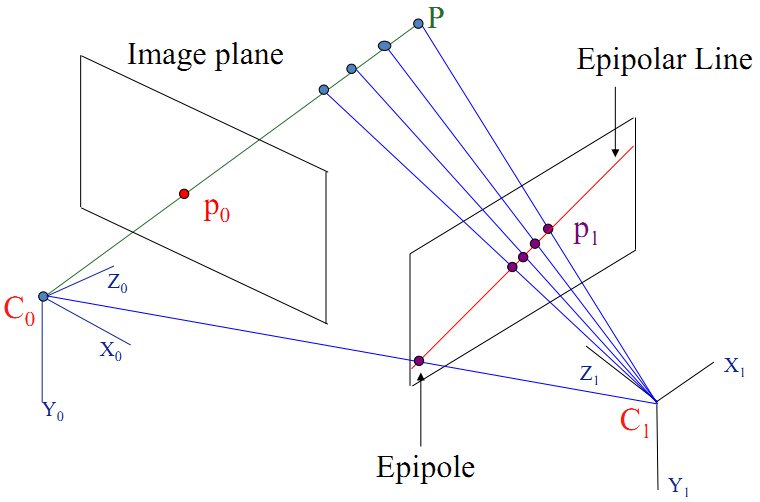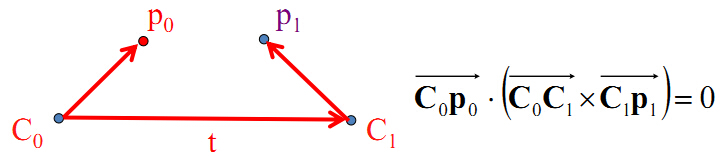p0 $p_{0}$在坐标系 C0 $C_{0}$中的表示,以及 p1 $p_{1}$在坐标系 C1 $C_{1}$中的表示为：
p0=x0y01c0 $p_{0} =\begin{pmatrix} x_{0}\\ y_{0}\\ 1 \end{pmatrix}_{c_{0}}$  和  p1=x1y11c1 $p_{1} =\begin{pmatrix} x_{1}\\ y_{1}\\ 1 \end{pmatrix}_{c_{1}}$

这里的 pi $p_{i}$是在摄像机坐标系 Ci $C_{i}$中的表示，而我们从图像平面得到的 (u,v,1)T $(u,v,1)^T$是在图像坐标系下的表示。我们知道从摄像机坐标系到图像坐标系，是左乘了一个内参数矩阵 K $K$。所以根据图像坐标中的(u,v)$(u,v)$得到其在摄像机坐标系下的坐标 p $p$需要左乘内参数的逆矩阵K1$K^{-1}$。在摄像机坐标系中的表示就称为normalized coordinates（hartley 书的第257页）。在程序中得到p的坐标代码演示如下：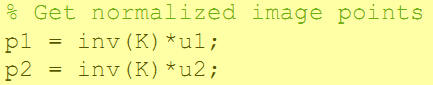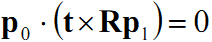(1)

一个向量a叉乘一个向量b可以表示为一个反对称矩阵乘以向量b的形式这时由向量a表示的反对称矩阵(skew symmetric matrix)如下: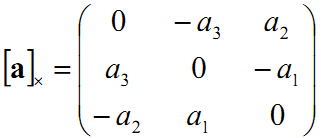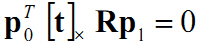本征矩阵 E 定义为 E=[t]×R $\color{Red}{E = [t]_{\times }R}$，它是一个3x3的矩阵：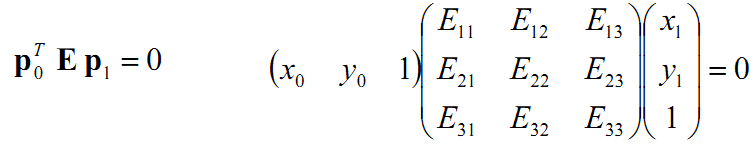一个3x3的矩阵是本征矩阵的充要条件是对它奇异值分解后，它有两个相等的奇异值，并且第三个奇异值为0。牢记这个性质，它在实际求解本征矩阵时有很重要的意义。这个性质的证明见Hartley的著作《Multiple view geometry》的第258页。

### 计算本征矩阵E、尺度scale的来由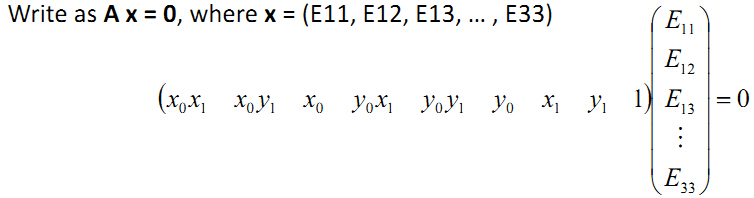(x0x1x0y1x0y0x1y0y1y0x1y11)E33E11/E33E12/E33E13/E33...1=0 $\begin{pmatrix} x_{0}x_{1} &x_{0}y_{1} &x_{0} &y_{0}x_{1} &y_{0}y_{1} &y_{0} &x_{1} &y_{1} &1 \end{pmatrix}E_{33}\begin{pmatrix} E_{11}/E_{33}\\ E_{12}/E_{33}\\ E_{13}/E_{33}\\ .\\ .\\ .\\ 1 \end{pmatrix}=0$

AX=0，x有8个未知量，需要A的秩等于8，所以至少需要8对匹配点。有了匹配点后，就只需要求解最小二乘问题了，上面这个方程的解就是矩阵A进行SVD分解 A=UΣVT $A=U\Sigma V^T$后，V矩阵最右边那一列的值。另外如果这些匹配点都在一个平面上那就会出现A的秩小于8的情况，这时会出现多解，会让你计算的E可能是错误的。上面是计算本征矩阵E的八点法，大家也可以去看看wiki的详细说明wiki链接
上面使用8点法计算E的过程使用的约束是 pT0Ep1=0 $p_0^TEp_1=0$。另外 E $E$这个矩阵它自身还有一个很棒的约束: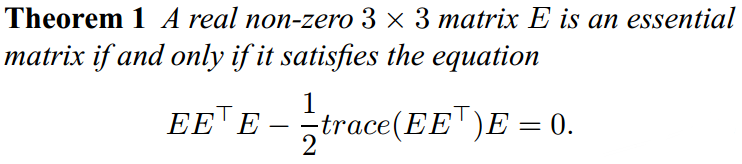然而在实际计算过程中，匹配点坐标存在误差，这会使得计算出的E可能不会满足之前提到的那条性质，所以我们需要把计算出的E投影到真正的本征矩阵空间，也就是使得它的三个奇异值中两个相等，一个为0。投影的方法如下，实际就是强制改变这个矩阵的奇异值，具体证明见YI Ma著作《An Invitation to 3D vision》的第86页。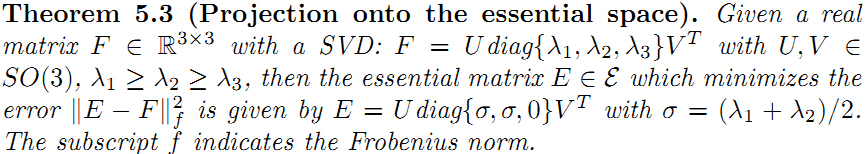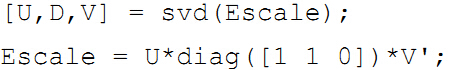### 特征点匹配

1. 计算特征点，然后计算特征描述子，通过描述子来进行匹配，优点准确度高，缺点是描述子计算量大。

### 从本征矩阵恢复R、T，尺度scale的进一步分析

我们知道本征矩阵 E 定义为 E=[t]×Rt^R$E = [t]_{\times }R或者\hat{t}R$。可以用下面的计算式从本征矩阵中恢复R和T， T^ $\hat{T}$表示T的反对称矩阵：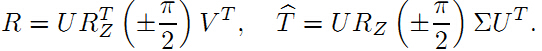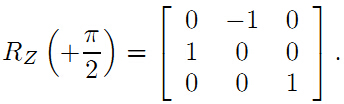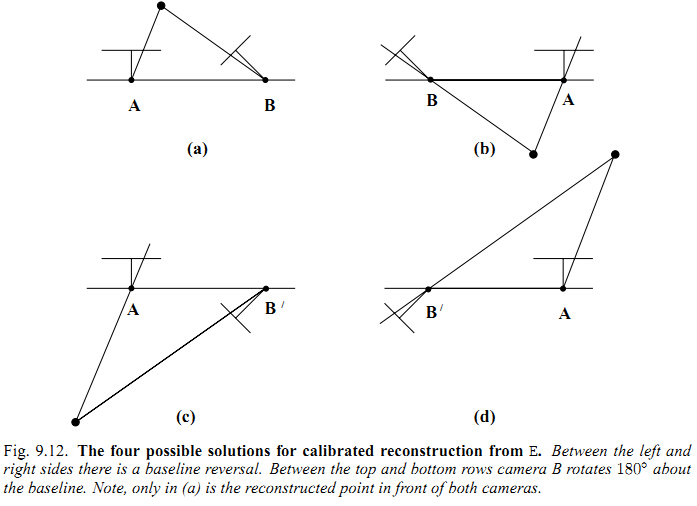这里我们有必要进一步的分析下尺度scale，从R，T的计算公式中，可以发现平移向量 t $t$E$E$的奇异值 Σ $\Sigma$有关。再回顾一下之前求 E $E$时候的方程以及提到的伸缩:
AE=AUΣV=0AsE=AUsΣV=0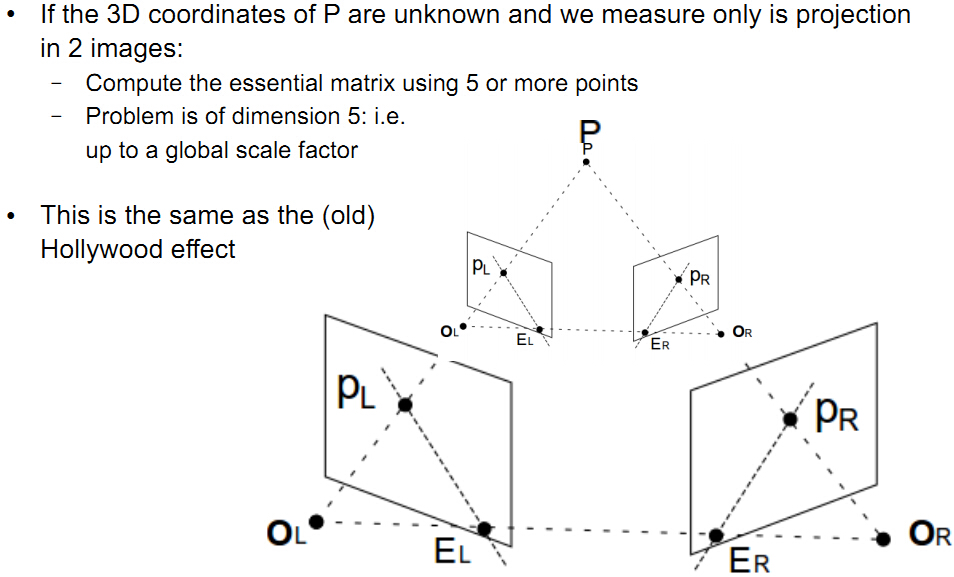这个图简单明了的演示了这种平移缩放作用。从图中也可以看出，由于尺度scale的关系，不同的t，决定了以后计算点P的深度也是不同的，所以恢复的物体深度也是跟尺度scale有关的，这就是论文中常说的结构恢复structure reconstruction，只是恢复了物体的结构框架，而不是实际意义的物体尺寸。并且要十分注意，每两对图像计算E并恢复R,T时，他们的尺度都不是一样的，本来是同一点，在不同尺寸下，深度不一样了，这时候地图map它最头痛了，所以这个尺度需要统一。
那么如何让scale之间统一呢？如果你一直采用这种2d-2d匹配计算位姿的方式，那每次计算的t都是在不同尺度下的，Davide Scaramuzza的论文《Visual odometry I》的computing the relative scale那部分讲了一种方法使得相邻位姿间的不同的尺度s经过缩放进行统一。我们已经知道出现尺度不一致是由于每次都是用这种计算本征矩阵的方式，而尺度就是在计算E时产生的。所以尺度统一的另一种思路就是后续的位姿估计我不用这种2d-2d计算本征 E <script type="math/tex" id="MathJax-Element-723">E</script>的方式了，也就说你通过最开始的两帧图像计算E恢复了R,T，并通过三角法计算出了深度，那我就有了场景点的3D坐标，后续的视频序列就可以通过3Dto2d(opencv里的solvePnp)来进行位姿估计，这样后续的视频序列就不用计算本征矩阵从而绕过了尺度，所以后续的视频序列的位姿和最开始的两帧的尺度是一样的了。但是，这样计算又会产生新的问题–scale drift。因为，两帧间的位姿总会出现误差，这些误差积累以后，使得尺度不再统一了，如下图所示(借用@清华大学王京同学的图，很容易帮助理解)：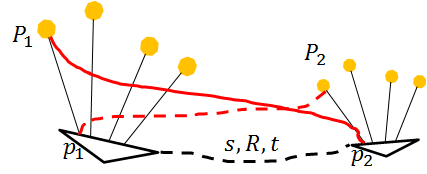相机的轨迹有了，接下来就是structure reconstruction 了，我们下篇博客见。
（转载请注明作者和出处： http://blog.csdn.net/heyijia0327 未经允许请勿用于商业用途）
reference：

01-03
09-28162402-241888
08-152008
06-122153
03-241万+
08-12
10-311052
10-151万+
07-055555
02-188956